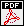## Local epsilon isomorphisms

Autors: David Loeffler, Otmar Venjakob and Sarah Zerbes
Titel:  Local epsilon isomorphisms
Jahr: 2013
Seiten: 52
In: Kyoto J. Math. 55 (2015), no. 1, 63--127.Abstract: In this paper, we prove the local $\varepsilon$-isomorphism'' conjecture of Fukaya and Kato \cite{fukayakato06} for a particular class of Galois modules obtained by tensoring a $\Zp$-lattice in a crystalline representation of $G_{\Qp}$ with a representation of an abelian quotient of $G_{\Qp}$ with values in a suitable $p$-adic local ring $R$. This can be regarded as a local analogue of the Iwasawa main conjecture for abelian $p$-adic Lie extensions of $\Qp$, extending earlier work of {\color{green} Kato for rank one modules (see \cite{venjakob11}) and of} Benois and Berger for the cyclotomic extension. We show that such an $\varepsilon$-isomorphism can be constructed using the Perrin-Riou regulator map, or its extension to the 2-variable case due to the first and third author.